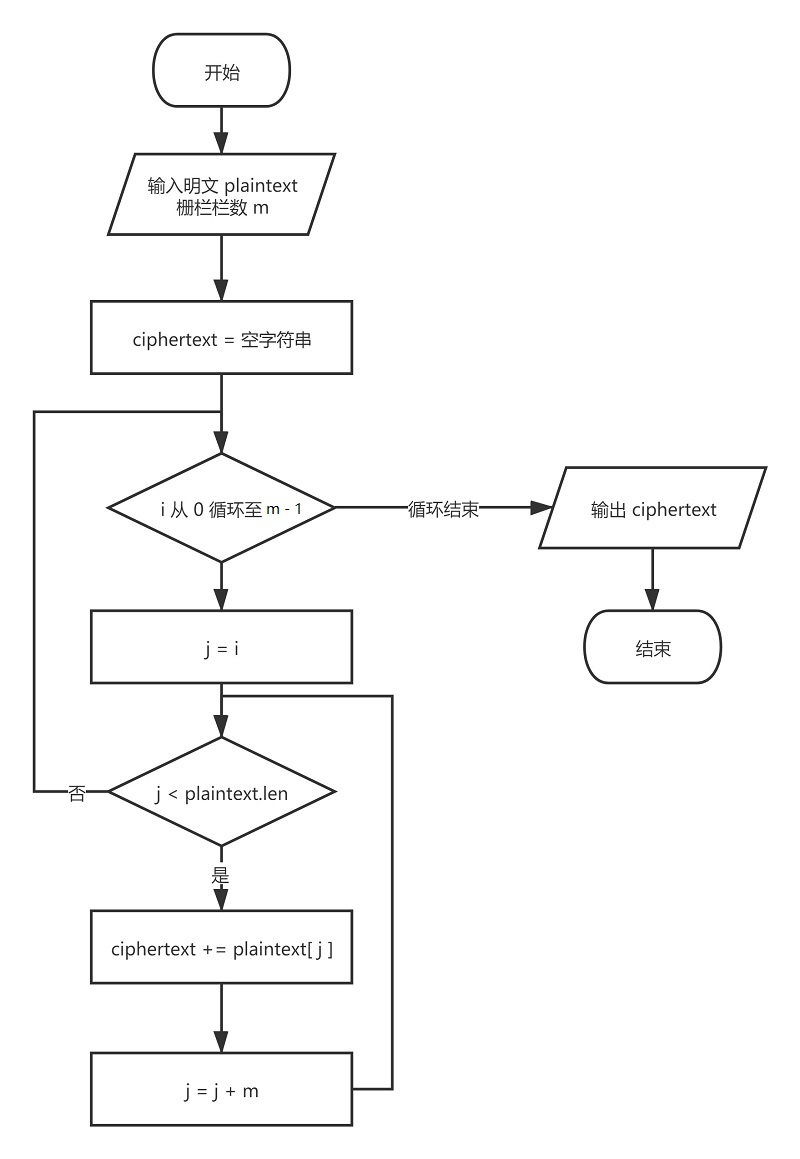# 1. 原理介绍

## 1.1 加密

(1) 首先将文本按如下方式排列，按列将文本排列成 m 行的栅栏

I e , L M
L Y Y o e
o o o v
v u u e

(2) 将文本按行取出，得到密文 Ie,LMLYYoeooovvuue## 1.2 解密

(1) 首先通过密文的长度 len = 18 和栅栏的栏数 m = 4 确定栅栏的列数 n；

(2) 将密文按行排列，重新生成 m 行 n 列的数组

I e , L M
L Y Y o e
o o o v
v u u e

(3) 将上述数组由上至下，由左至右读取，恢复明文 ILoveYou,YouLoveMe

(1) 计算出 n = 5，r = 2，mid = (m - r) · n = 10, Len = 18

(1) 计算出 n = 5，r = 2，mid = (m - (m · n - Len)) · n = 10, Len = 18. (--2023-11-24更新, 感谢评论区SojournWayfarer和nanximuzi指出的错误--)

(2) 前 mid 个密文以 5 为单位分组，后 Len - mid = 8 个密文以 n - 1 = 4 为单位分组，得到

I L o v
e Y o u
, Y o u
L o v e
M e

(3) 按照加密的方式读取分组，得到明文

# 2. 具体实现(python)

## 2.1 加密

def encrypt(plaintext: str, m: int):
"""
:param plaintext: 明文
:param m: 栏数
:return: 密文
"""
ciphertext, length = [], len(plaintext)
for i in range(m):  # 取出每个分组第 i 个元素
for j in range(i, length, m):
ciphertext.append(plaintext[j])
return ''.join(ciphertext)


## 2.2 解密

def decrypt(ciphertext: str, m: int):
"""
:param ciphertext: 密文
:param m: 栏数
:return: 明文
"""
plaintext, length = [], len(ciphertext)
q, r = divmod(length, m)
n = (q + 1) if r else q  # 计算 n
# mid = n * (m - r) # 感谢SojournWayfarer和nanximuzi指出的错误
mid = n * (m - (m * n - length))
for i in range(n - 1):
# 前 m-r 个分组
for j in range(i, mid, n):
plaintext.append(ciphertext[j])
# 后 r 个分组
for j in range(mid + i, length, n - 1):
plaintext.append(ciphertext[j])
# 前 m-r 个分组最后一个元素
for j in range(n - 1, mid, n):
plaintext.append(ciphertext[j])
return ''.join(plaintext)


## 2.3 测试样例及结果

# 样例一
plaintext = "whateverisworthdoingisworthdoingwell"
m = 3 # 栅栏行数
ciphertext = "wtesrdnsrdneherwtogwtoglaviohiiohiwl"

# 样例二
plaintext = "healthismoreimportantthanwealth"
m = 2
ciphertext = "hatimriprathnelhelhsoemotntawat"


# 3. 其它

posted @ 2021-07-12 22:52  kentle  阅读(2572)  评论(3编辑  收藏  举报